This website works best with JavaScript enabled. Learn how to enable JavaScript.

# How Does VWO Calculate the Chance to Beat

Last updated

The Chance to Beat All (CTBA) represents the probability that a variation will perform better than the control version of your website or mobile app. For example, a chance of 60% means that the variation is likely to perform better than the control 60% of the time. This value also means that there is a 40% chance that your results are completely due to a random chance.

ATTENTION Currently, this method of calculating the CTBA is only used for Multivariate tests and Personalization, because they still follow the Frequentist method of calculating a winner. To determine the winning variation in A/B and Split URL tests  we follow the SmartStats (Bayesian) method of calculating the chance to beat all versions.

The success or failure of a variation is calculated by comparing the results against a base version (by default, the control page). You can change the base for your test at any time.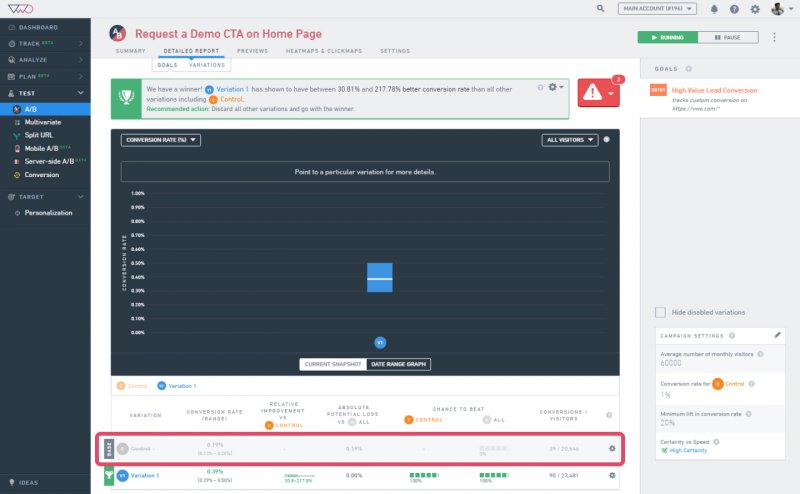Please note that VWO calculates chance to beat on the basis of the test threshold settings you have defined in Settings > Campaign Settings.To determine the chance to beat of a variation, VWO calculates the:

• Conversion rates of the control and variation
• Standard error for the control and variation
• Chance to beat for a variation

### Calculating Conversion Rates of the Control and Variations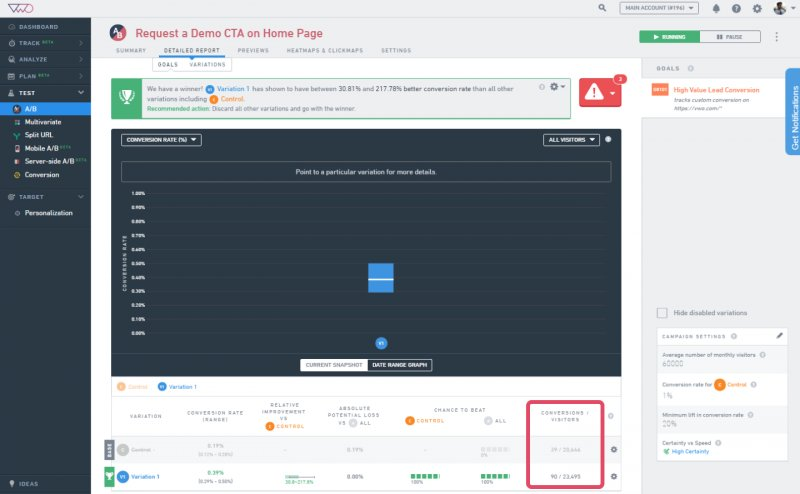Mathematically, the conversion rate is a binomial random variable, that is, it can have two possible values. It is represented as the variable p. In A/B testing, you can calculate the value of p by observing n visitors on the webpage that is being tested. From those n visitors, you can calculate the number of conversions. The percentage value from the result is the conversion rate of your website or app.

Conversion rate (p) = Actual conversions/Visits to the website

The percentage value is the conversion rate of your website.

For example, consider that you are running a test to review the new design of your landing page. The control receives 894 visits of which 423 are converted. On the other hand, the variation receives 863 visits of which 458 are converted. These values imply that the conversion percentage value of the control is 47% and that of the variation is 53%.

### Calculating the Standard Error for the Control and Variations

If you repeat the same test multiple times, it is likely that you will get a different value of p (or conversion rate of a variation) every time. This happens because in statistical terms, we’re sampling and like every sample, there’s a range of error associated with it (because it does not cover the entire population). To avoid repeated tests, we can use the already established statistical formulas to calculate the standard error to determine how much deviation from the average conversion rate (p) can be expected from the test results. The deviation is the range within which the conversion rate is usually found. Smaller the deviation, more confident you can be about estimating the true conversion rate. For a given conversion rate (p) and number of trials (n), standard error is calculated as:

Standard Error (SE) = Square root of (p * (1-p) / n)

In the example, the SE for control = Square root of (0.4732*(1-0.473/894) = 1.67 x 10-2

And, the SE for variation = Square root of (0.5307*(1-0.5307/863) =1.70 x 10-2

(Please note that the percentage values are converted to decimal in the above calculations).

To get 95% range for the conversion rate, multiply the standard error value by 2 (or 1.96 to be precise). You can be sure with 95% confidence that your true conversion rate lies within this range: p% ± 2 * SE.

In VWO, we calculate the conversion rate range for 80%, not 95%. Hence, we multiply the SE value by 1.28.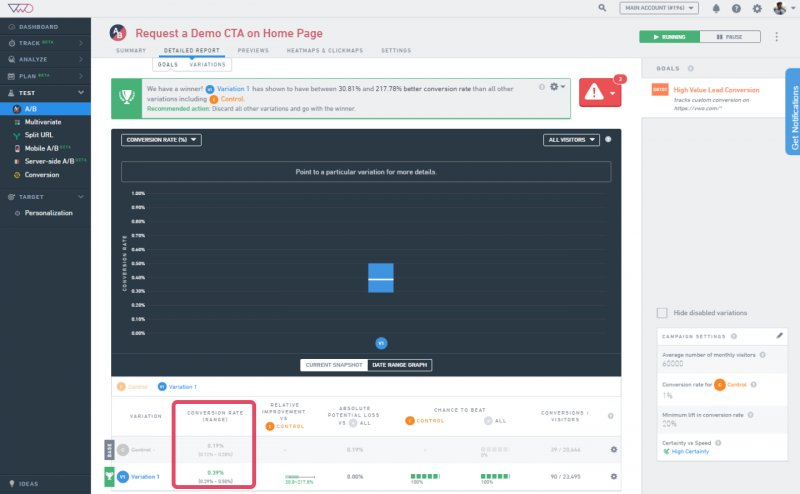In the example discussed above, the standard error for the control is 1.67, while that of the variation is 1.70. Next, multiply the values by 1.28. The conversion rate range thus calculated is ± 2.14% for control and ± 2.17% for the variation.

### Calculating the CTBA for a Variation

To determine chance to beat for a variation, we calculate the Z-score for control and variation. A Z-score is used in statistics to model any normal distribution as a standard normal distribution.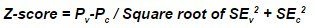Where,

• Pv = Conversion rate of variation
• Pc = Conversion rate of control
• SEv = Standard error of variation
• SEc = Standard error of control

For our example discussed above, the Z-score can be derived as follows:

Z-score = (0.53 – 0.47)/Square root of 0.01698882 + 0.0166982 = 2.41605

After the Z-score is calculated, VWO calculates standard normal distribution to derive the chance percentage for the variation. You can refer to the following normal distribution table to understand how CTBA is derived for a variation: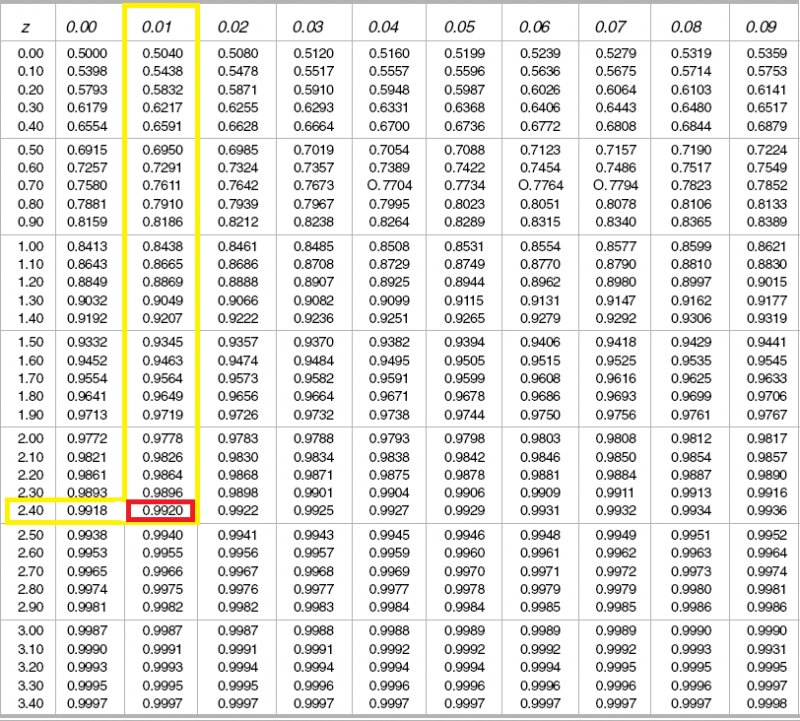As we see, for our z-score of 2.41, the normal distribution value is approximately 99%.